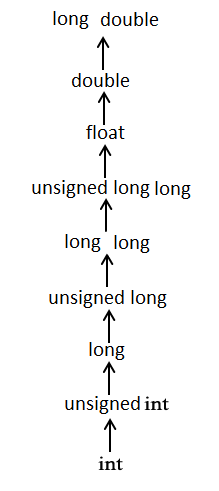# 数据类型转换

C 语言中如果一个表达式中含有不同类型的常量和变量，在计算时，会将它们自动转换为同一种类型。此外，在 C 语言中也可以对数据类型进行强制转换。

## 隐式（自动）类型转换

• 浮点数赋给整型，该浮点数小数被舍去；
• 整数赋给浮点型，数值不变，但是被存储到相应的浮点型变量中；
#include <stdio.h>

int main(){
int  i = 17;
char c = 'c'; /* ascii 值是 99 */
int sum;

sum = i + c;
printf("Value of sum : %d\n", sum );

}


### 常用的算术转换## 显式（强制）类型转换

#include <stdio.h>

int main() {
float f, a, x=3.6, y=5.2;
int i=4, b;
a = x + y;
b = (int)(x + y);
f = 10 / i;

printf("a=%d, b=%d, f=%f, x=%f\n", a, b, f, x);
return 0;
}


$./main a=8.799999, b=8, f=2.000000, x=3.600000  #include <stdio.h> int main() { int sum = 17, count = 5; double mean; mean = (double)sum / count; // mean = (double)(sum / count); 此时返回 3.000000 printf("Value of mean: %f", mean); return 0; }  需要注意的是，强制类型转换运算符的优先级大于除法，因此 sum 的值首先被转换为 double 型，然后除以 count，得到一个类型为 double 的值。注意，如果是 (double)(sum / count) 的话，则会先进行整数运算得到 3 再类型转换为双精度浮点数 3.000000。 运行： $ ./main
Value of mean: 3.400000


# 字符串转换为数字类型：atoi、atol、atof

C 语言的 stdlib.h 提供了 atoi、atol、atof 函数，用于将字符串类型变量转换为 int、long、float 类型的数字。

• atoi 会扫描参数 nptr 字符串，跳过前面的空格字符，直到遇上数字或正负符号才开始做转换，而再遇到非数字或字符串结束时（e.g. ‘\0’）才结束转换，并返回整型数。否则，返回零。
int atoi(const char *nptr);

• atol 字符串类型转换为 long 类型，同上。
long atol(const char *nptr);

• atof 字符串类型转换为 float 类型。atof 会扫描参数字符串，跳过前面的空格字符，直到遇上数字或正负符号才开始做转换，而再遇到非数字或字符串结束时（e.g. ‘\0’）才结束转换，并将结果返回。参数 nptr 字符串可包含正负号、小数点或 E/e 来表示指数部分，如：123.456 或 123e-2。返回值是转换后的浮点型数。
double atof(const char *nptr);


#include <stdlib.h>
#include <stdio.h>

int main(void)
{
int n;
char *str = "23.5";
n = atoi(str);
printf("string=%s,n=%d\n", str, n);
return 0;
}


#### C语言入门到高阶--字符串、类型转换与运算符

06-23

11-084万+

#### C/C++数据类型转换小结04-28190

#### 数据格式转换

08-292万+

#### 青少儿编程NOIP信息学奥赛通关必备-C++语法专题-数据类型转换

02-27

11-152万+

#### C语言中强制数据类型转换

04-013418

#### C语言的数据的强制类型转换

06-25569

#### C 语言编程 — 结构体的数据类型转换©️2020 CSDN 皮肤主题: 编程工作室 设计师: CSDN官方博客点击重新获取扫码支付1.余额是钱包充值的虚拟货币，按照1:1的比例进行支付金额的抵扣。
2.余额无法直接购买下载，可以购买VIP、C币套餐、付费专栏及课程。余额充值